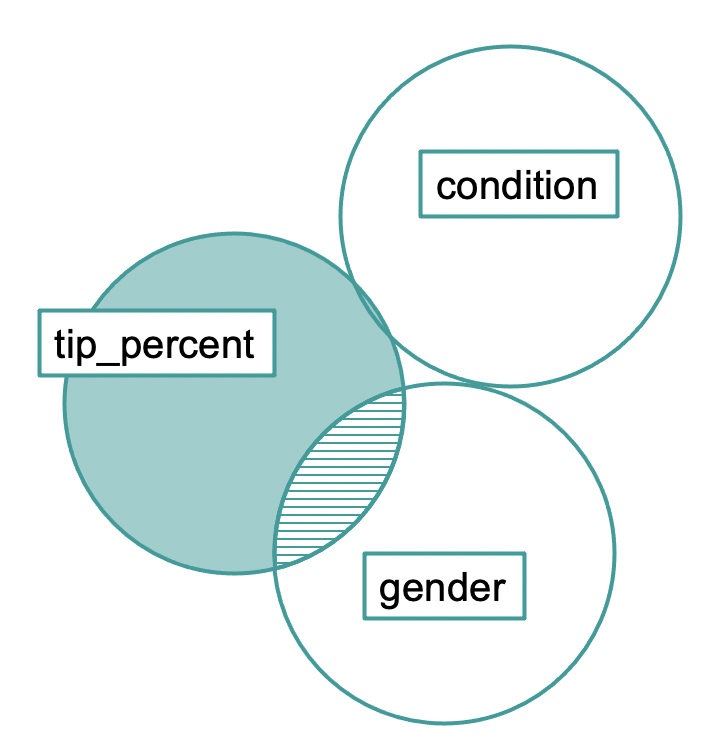## Course Outline

• segmentGetting Started (Don't Skip This Part)
• segmentStatistics and Data Science: A Modeling Approach
• segmentPART I: EXPLORING VARIATION
• segmentChapter 1 - Welcome to Statistics: A Modeling Approach
• segmentChapter 2 - Understanding Data
• segmentChapter 3 - Examining Distributions
• segmentChapter 4 - Explaining Variation
• segmentPART II: MODELING VARIATION
• segmentChapter 5 - A Simple Model
• segmentChapter 6 - Quantifying Error
• segmentChapter 7 - Adding an Explanatory Variable to the Model
• segmentChapter 8 - Models with a Quantitative Explanatory Variable
• segmentPART III: EVALUATING MODELS
• segmentChapter 9 - The Logic of Inference
• segmentChapter 10 - Model Comparison with F
• segmentChapter 11 - Parameter Estimation and Confidence Intervals
• segmentPART IV: MULTIVARIATE MODELS
• segmentChapter 12 - Introduction to Multivariate Models
• segmentChapter 13 - Multivariate Model Comparisons
• segmentFinishing Up (Don't Skip This Part!)
• segmentResources

### list Statistics and Data Science: A Modeling Approach

Book
• College / Advanced Statistics and Data Science (ABCD)
• College / Statistics and Data Science (ABC)
• High School / Advanced Statistics and Data Science I (ABC)
• High School / Statistics and Data Science I (AB)
• High School / Statistics and Data Science II (XCD)

## 13.9 Error and Inference from Models with Multiple Categorical Predictors

### The ANOVA Table for the tip_percent ~ condition + gender Model

Let’s take a look at the ANOVA table to see how much error has been reduced (or, explained) by the multivariate model and how much each predictor uniquely contributes to this overall model.

require(coursekata) # here is the code to find the best fitting model # modify this to generate the ANOVA table for this model lm(tip_percent ~ condition + gender, data = tip_exp)  supernova(lm(tip_percent ~ condition + gender, data = tip_exp)) # students may also save the model and then run supernova so we might just want to check for output #multi_model <- lm(tip_percent ~ condition + gender, data = tip_exp) #supernova(multi_model) # temporary SCT ex() %>% check_error()
CK Code: D2_Code_Error_01
Analysis of Variance Table (Type III SS)
Model: tip_percent ~ condition + gender

SS df       MS      F    PRE     p
--------- --------------- | --------- -- -------- ------ ------ -----
Model (error reduced) |  2534.538  2 1267.269 12.667 0.2275 .0000
condition                 |    12.154  1   12.154  0.121 0.0014 .7283
gender                 |  2531.353  1 2531.353 25.302 0.2273 .0000
Error (from model)    |  8603.860 86  100.045
--------- --------------- | --------- -- -------- ------ ------ -----
Total (empty model)   | 11138.398 88  126.573   

We can represent this result in the Venn diagram below. The condition variable overlaps hardly at all with either tip_percent or gender.### Interpreting the p-values

The p-values in the ANOVA table can help us compare different possible models of the DGP.

Analysis of Variance Table (Type III SS)
Model: tip_percent ~ condition + gender

SS df       MS      F    PRE     p
--------- --------------- | --------- -- -------- ------ ------ -----
Model (error reduced) |  2534.538  2 1267.269 12.667 0.2275 .0000
condition                 |    12.154  1   12.154  0.121 0.0014 .7283
gender                 |  2531.353  1 2531.353 25.302 0.2273 .0000
Error (from model)    |  8603.860 86  100.045
--------- --------------- | --------- -- -------- ------ ------ -----
Total (empty model)   | 11138.398 88  126.573


The p-value for condition (.73) means that the F ratio for condition in the multivariate model could easily have been generated just by random chance, even if the true effect of condition in the DGP were actually equal to 0. We therefore would not reject the simple model (tip_percent ~ gender) being compared to the multivariate model.

The p-value for gender (.0001) implies a different story. It says that there is a less than .0001 chance that the F for gender would have resulted from a DGP in which the effect of gender is equal to 0. We can reject a model, therefore, that does not include gender (in this case, the model tip_percent ~ condition).

### Selecting a Model of the DGP

Based on what we have learned from the ANOVA table, it seems reasonable to arrive at a final model of tip_percent ~ gender. Before finalizing our decision, we can compare the parameter estimates and ANOVA tables for the multivariate and gender models.

The two ANOVA tables look like this:


Model: tip_percent ~ condition + gender

SS df       MS      F    PRE     p
--------- --------------- | --------- -- -------- ------ ------ -----
Model (error reduced) |  2534.538  2 1267.269 12.667 0.2275 .0000
condition                 |    12.154  1   12.154  0.121 0.0014 .7283
gender                 |  2531.353  1 2531.353 25.302 0.2273 .0000
Error (from model)    |  8603.860 86  100.045
--------- --------------- | --------- -- -------- ------ ------ -----
Total (empty model)   | 11138.398 88  126.573


Model: tip_percent ~ gender

SS df       MS      F    PRE     p
----- --------------- | --------- -- -------- ------ ------ -----
Model (error reduced) |  2522.384  1 2522.384 25.470 0.2265 .0000
Error (from model)    |  8616.014 87   99.035
----- --------------- | --------- -- -------- ------ ------ -----
Total (empty model)   | 11138.398 88  126.573


When we look at the confidence intervals around the parameter estimates for gendermale (the change in prediction if the table had a male server), we see that they are similar between the single parameter model and the multivariate model (somewhere between -15 and -6.5).

confint(gender_model) confint(multi_model)
                 2.5 %    97.5 % (Intercept)  27.83938 33.736615 gendermale  -14.84160 -6.454402                           2.5 %    97.5 % (Intercept)           26.815575 34.037313 conditionsmiley face  -3.478493  4.957586 gendermale           -14.891065 -6.454986

Both models estimate that male servers will get lower tip percentages. The fact that the parameter estimates between the models don’t change very much reflects the fact that there is very little redundancy between condition and gender.